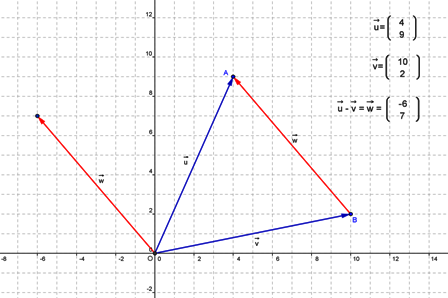The subtraction of two vector is represented by the "other" diagonal (see adding 2D vectors and adding vectors) of the parallelogram constructed using the vectors as the sides.

In general, subtraction is accomplished by adding the negative, so we can think of computing $u-v$ as being $u+(-v).$

If you can add quantities, and negate quantities, then you can subtract.(none) (none) (none)
(none) (none)

## You are here

VectorSubtraction